International
Tables for
Crystallography
Volume B
Reciprocal space
Edited by U. Shmueli

International Tables for Crystallography (2010). Vol. B, ch. 2.5, pp. 383-385

## Section 2.5.7.9. Refinement of a 3D structure

P. A. Penczekg

#### 2.5.7.9. Refinement of a 3D structure

| top | pdf |

Given an initial low-resolution model of the 3D structure and the data set of 2D projection images of the complex that have Fourier-space information extending beyond the resolution of the model, it is possible to refine the structure such that the full extent of the resolution information in the data will be utilized. In some cases, it is also possible to use as an initial structure in the refinement procedure a structure of a homologous protein, thus avoiding the process of ab initio structure determination altogether. The goal of the refinement is to find such orientation parameters for each of the particle projections for which (2.5.7.6)is minimized. There exist various implementations of the structure-refinement strategy and they can be roughly divided into those that perform exhaustive searches for all five orientation parameters (two translations and three Eulerian angles per 2D projection image) and those that perform local searches, usually by employing gradient information. Finally, the strategies may differ in how the correction for the CTF is implemented.

The original 3D projection-matching strategy (Penczek et al., 1994) is based on the observation that given an ideal structure f and the necessary parameters of the CTF and image-formation model, it is straightforward to find five orientation parameters for each projection image. One begins with the determination of the sufficient angular step: assuming the structure is properly sampled at the Nyquist frequency and has a real-space radius of r voxels, the angular step is given by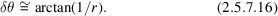Next, keeping in mind that projection directions are parametrized by two Eulerian angles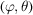, one generates a set of projection directions quasi-uniformly distributed over half a unit sphere (or, in the case of a symmetric structure, over an asymmetric subunit) by taking fixed steps along the altitude or tilt angleand a number of samples azimuthally in proportion to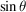(Penczek et al., 1994). So, for a chosen constant increment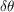and givenangle the increment of theangle varies according to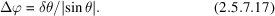If all three Eulerian angles are to be sampled, as is necessary in some applications, then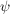is sampled uniformly in steps of.

In order to find the orientation parameters of projection images, one step of projection matching is performed. The reference structure is projected in all directions given by (2.5.7.17), yielding a set of reference images. Next, for each projection image, 2D cross-correlation functions with all reference images are calculated using one of the methods described in Section 2.5.7.6and the overall maximum yields the translation, the in-plane rotation angle, the number of the most similar reference image (thus the remaining two Eulerian angles) and information about whether the image should be mirrored. Given this, a new 3D structure can be calculated using a 3D reconstruction algorithm (see Section 2.5.6). This simple protocol constitutes the core of 3D projection alignment (Fig. 2.5.7.4).Figure 2.5.7.4 | top | pdf |Schematic of the 3D projection-alignment procedure.

In a simple implementation of the 3D projection-matching procedure, all projection data are assembled into defocus groups, i.e., groups of projection images that have similar defocus settings (Frank et al., 2000). During refinement, for each defocus group the reference volume is multiplied by the CTF with the appropriate defocus value, one step of projection matching is performed and a refined structure is reconstructed for this group (Fig. 2.5.7.5). In addition, the within-group resolution is estimated using the Fourier shell correlation (FSC) approach (2.5.7.19)applied to two volumes calculated from two subsets of projection images randomly split into halves. After all defocus groups have been processed, the individual refined volumes are merged in Fourier space with a CTF correction using Wiener-filter methodology (Penczek et al., 1997),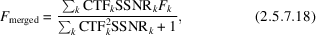where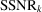is the spectral signal-to-noise ratio estimated for each defocus group using (2.5.7.22). Subsequently, the resolution of the merged volume is estimated by merging the half-volumes into two half-merged volumes using (2.5.7.18)and comparing them using (2.5.7.19). Next, the merged volume is filtered using (2.5.7.25)and the structure is centred so that its centre of mass is placed at the centre of the volume in which it is embedded.Figure 2.5.7.5 | top | pdf |Schematic of 3D projection alignment with CTF correction performed on the level of 3D maps reconstructed from projection images sorted into groups that share similar defocus settings.

The 3D projection-matching approach works very well during the initial stages of the refinement as it constitutes a very efficient approach to an exhaustive search for orientation parameters of all projection data. Once the orientation parameters are known to a degree of accuracy, it is straightforward to modify the procedure such that only subsets of reference projections are generated at a time and projection images are compared only with reference projections within a specified angular distance from their angular direction established during previous iteration. This modification speeds up the procedure significantly and makes it possible to refine structures to very high resolution by using a very small angular step. Another possible modification is to introduce an additional step of 2D alignment of the projection data that share the same angular direction (Ludtke et al., 1999). The advantage is that this can correct possible errors of alignment to the projection of a limited-resolution reference structure and also, to an extent, reduces the danger of bias from artifacts in the reference structure. Finally, it is also possible to incorporate into the refinement strategy a correction for the envelope function of the microscope (Ludtke et al., 1999). The 3D projection-matching strategy is widely popular and most EM software packages have implementations of various versions of basic strategies, as outlined above (Frank et al., 1996; Ludtke et al., 1999; Hohn et al., 2007).

A possible improvement over the 3D projection-matching procedure can be achieved by working in transformed spaces in which the distinction between orientation search and 3D reconstruction is removed: (1) spherical harmonics (Provencher & Vogel, 1988; Vogel & Provencher, 1988), which have found applications exclusively in the determination of icosahedral structures (Yin et al., 2001, 2003); (2) Radon transform (Radermacher, 1994), with selected applications in the determination of asymmetric particles (Ruiz et al., 2003); or (3) Fourier transform, implemented in the FREALIGN package (Grigorieff, 2007). In FREALIGN, the transformation between the arbitrarily oriented Fourier 2D central section and the 3D Fourier Euclidean grid is implemented using trilinear interpolation that includes ad hoc correction for the CTF effects. In high-resolution structure-refinement mode, the program uses a gradient-based Powell optimization algorithm (Powell, 1973), thus overcoming the main deficiency of 3D projection-matching algorithms.

A unified approach to direct minimization of (2.5.7.6)was proposed by Yang et al. (2005) and is implemented in the SPARX package as the YNP method (Hohn et al., 2007). The premise of the YNP method is that the orientation parameters are approximately known (thus the initial 3D map) and both the orientation parameters and the density map are updated simultaneously in a gradient-based optimization scheme. In the YNP method, the derivatives with respect to the density distribution are calculated analytically and the derivatives with respect to orientation parameters are calculated using finite difference approximations. The YNP method is very efficient and its major advantage is that it avoids many problems associated with approximate solutions inherent in methods that work in transform spaces. The projection/backprojection operations are carried out rapidly using linear interpolation, which due to sufficient oversampling of the data does not have a significant adverse impact on the solution. Moreover, because the density map f is updated simultaneously with the orientation parameters, the computationally demanding separate step of 3D reconstruction is eliminated.

### References

Frank, J., Penczek, P., Agrawal, R. K., Grassucci, R. A. & Heagle, A. B. (2000). Three-dimensional cryoelectron microscopy of ribosomes. Methods Enzymol. 317, 276–291.
Frank, J., Radermacher, M., Penczek, P., Zhu, J., Li, Y., Ladjadj, M. & Leith, A. (1996). SPIDER and WEB: processing and visualization of images in 3D electron microscopy and related fields. J. Struct. Biol. 116, 190–199.
Grigorieff, N. (2007). FREALIGN: High-resolution refinement of single particle structures. J. Struct. Biol. 157, 117.
Hohn, M., Tang, G., Goodyear, G., Baldwin, P. R., Huang, Z., Penczek, P. A., Yang, C., Glaeser, R. M., Adams, P. D. & Ludtke, S. J. (2007). SPARX, a new environment for cryo-EM image processing. J. Struct. Biol. 157, 47–55.
Ludtke, S. J., Baldwin, P. R. & Chiu, W. (1999). EMAN: semiautomated software for high-resolution single-particle reconstructions. J. Struct. Biol. 128, 82–97.
Penczek, P. A., Grassucci, R. A. & Frank, J. (1994). The ribosome at improved resolution: new techniques for merging and orientation refinement in 3D cryo-electron microscopy of biological particles. Ultramicroscopy, 53, 251–270.
Penczek, P. A., Zhu, J., Schröder, R. & Frank, J. (1997). Three-dimensional reconstruction with contrast transfer function compensation from defocus series. Scan. Microsc. Suppl. 11, 1–10.
Powell, M. J. D. (1973). On search directions for minimization algorithm. Math. Program. 4, 193–201.
Provencher, S. W. & Vogel, R. H. (1988). Three-dimensional reconstruction from electron micrographs of disordered specimens. I. Method. Ultramicroscopy, 25, 209–221.
Radermacher, M. (1994). Three-dimensional reconstruction from random projections: orientational alignment via Radon transforms. Ultramicroscopy, 53, 121–136.
Ruiz, T., Mechin, I., Bar, J., Rypniewski, W., Kopperschlager, G. & Radermacher, M. (2003). The 10.8-A structure of Saccharomyces cerevisiae phosphofructokinase determined by cryoelectron microscopy: localization of the putative fructose 6-phosphate binding sites. J. Struct. Biol. 143, 124–134.
Vogel, R. H. & Provencher, S. W. (1988). Three-dimensional reconstruction from electron micrographs of disordered specimens. II. Implementation and results. Ultramicroscopy, 25, 223–239.
Yang, C., Ng, E. G. & Penczek, P. A. (2005). Unified 3-D structure and projection orientation refinement using quasi-Newton algorithm. J. Struct. Biol. 149, 53–64.
Yin, Z. H., Zheng, Y. L., Doerschuk, P. C., Natarajan, P. & Johnson, J. E. (2003). A statistical approach to computer processing of cryo-electron microscope images: virion classification and 3-D reconstruction. J. Struct. Biol. 144, 24–50.
Yin, Z. Y., Zheng, Y. L. & Doerschuk, P. C. (2001). An ab initio algorithm for low-resolution 3-D reconstructions from cryoelectron microscopy images. J. Struct. Biol. 133, 132–142.